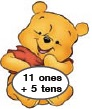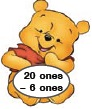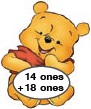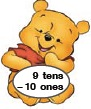# User ForumSubject :IMO    Class : Class 1

Which of the following shows the least value?

(a)(b)(c)(d)B

## Ans 1: (Master Answer)

Class : Class 1

(B):

A. 11 ones + 5 tens = 11 + 50 = 61

B. 20 ones - 6 ones = 20 - 6 = 14

C. 14 ones + 18 ones = 14 + 18 = 32

D. 9 tens - 10 ones = 90 - 10 = 80

Therefore , 14 is the least value among all.